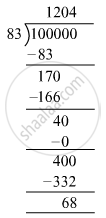# Find the least 6-digit number exactly divisible by 83. - Mathematics

Fill in the Blanks

Find the least 6-digit number exactly divisible by 83.

#### Solution

Least six-digit number = 100000
Here, dividend = 100000 and divisor = 83In order to find the least 6-digit number exactly divisible by 83, we have to add 83 − 68 = 15 to the dividend.
I.e., 100000 + 15 = 100015
So, 100015 is the least six-digit number exactly divisible by 83.

Is there an error in this question or solution?

#### APPEARS IN

RS Aggarwal Class 6 Mathematics
Chapter 3 Whole Numbers
Exercise 3E | Q 12 | Page 56